GRE Subject Test: Chemistry : Enthalpy

Example Questions

Example Question #1 : Physical Chemistry

Propane gas combusts according the following chemical equation: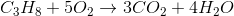Given the following standard enthalpy values, what is the enthalpy of the reaction for the combustion of one mole of propane?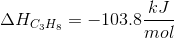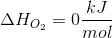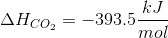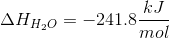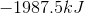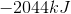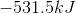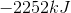Explanation:

Given the molar enthalpy values for reactants and products, we can solve for the enthalpy of the reaction using the equation: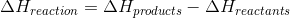Keep in mind that the molar enthalpy values must be multiplied by the coefficients that are present in the balanced reaction: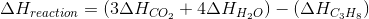Oxygen is omitted because its enthalpy value is zero.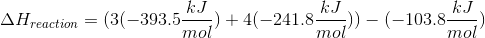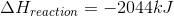Example Question #2 : Physical Chemistry

Which is not characteristic of an endothermic reaction?

The net change of enthalpy is positive

Absorption of energy from surroundings

Breaking of a chemical bond

A chemical reaction that feels cold

Releasing of energy to surroundings

Releasing of energy to surroundings

Explanation:

An endothermic reaction absorbs energy in the form of heat. An endothermic reaction involves breaking of chemical bonds because it involves the absorption of energy. Endothermic reactions tend to feel cold because it is taking heat away from your skin. Since endothermic reactions involve absorbing energy, often in the form of heat, the change in enthalpy is positive.

Therefore, the answer is "releasing of energy to surroundings" which is not a characteristic of an endothermic process. Instead, it is a characteristic of an exothermic reaction which is the direct opposite of an endothermic reaction.

Example Question #1 : Enthalpy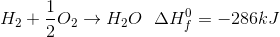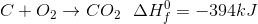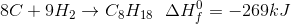Given the enthalpies of formation, what is the enthalpy of combustion of octane in the reaction: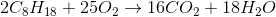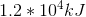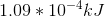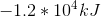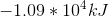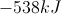Explanation:

The equation for enthalpy of reaction is: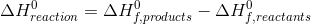Given our chemical reaction and the enthalpies of formation, we can find the enthalpy of reaction.First, find the total enthalpy for the products.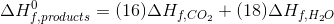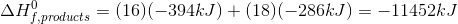Then, find the total enthalpy for the reactants.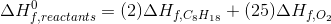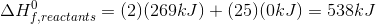Since the oxygen is elemental, its heat of formation is zero.

Return to the original equation to calculate the final enthalpy of reaction.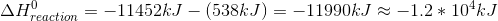All GRE Subject Test: Chemistry Resources